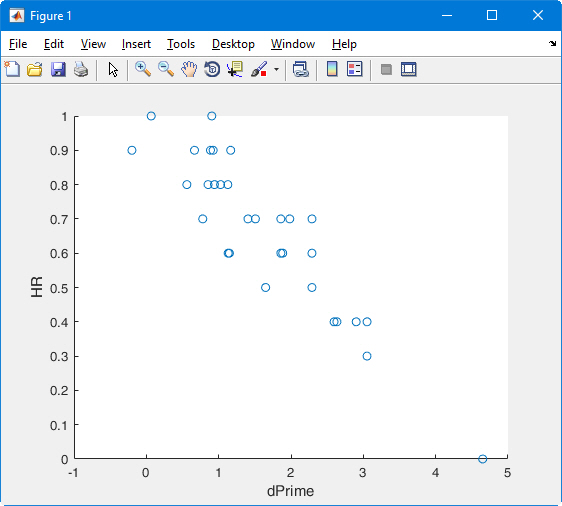# #StackBounty: #matlab #signal-detection Negative correlation between d' and hit rates?

### Bounty: 100

I have data from a relatively easy task where subjects had to detect a signal in noise. I computed the hit and false alarm rates (HR, FAR), and then proceeded to compute d’ using the standard formula, found e.g. in Green&Swets 1966, and described also here:

``````d' = z(FAR) – z(HR),
``````

where the z-scores for the left-tail p-values from the normal distribution are computed in Matlab as:

``````z(HR)  = norminv(1-HR, 0, 1)
z(FAR) = norminv(1-FAR, 0, 1).
``````

This produced all-negative values for d’, and I know the usual practice is to then inverse the sign in the d’ formula, to get positive d’ values:

``````d' = z(HR) – z(FAR)
``````

As a sanity check, I created a cross-subjects scatterplot of raw HR values against d’, expecting to find a by-and-large positive correlation. However, what I found was a very neat negative correlation:I know the p-value in the above formulas for the z-scores are taken by some not as `1-p` but simply as `p`, thus I recalculated z(HR) and z(FAR) as

``````z(HR)  = norminv(HR, 0, 1)
z(FAR) = norminv(FAR, 0, 1)
``````

To have positive d’ values, I went back to its `z(FAR)-z(HR)` formula, but the values & their plot end up looking the same as the screenshot above.

Surely, assuming moderate FARs across the board, subjects with the highest HRs should in principle also have the highest d’ values?! What am I getting confused here?

REFERENCE: Green, D. M., & Swets, J. A. (1966). Signal detection theory and psychophysics (Vol. 1). Wiley New York.

Get this bounty!!!

This site uses Akismet to reduce spam. Learn how your comment data is processed.